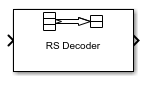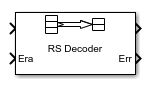# Integer-Output RS Decoder

Decode Reed-Solomon code to recover integer vector data

•Libraries:
Communications Toolbox / Error Detection and Correction / Block

## Description

The Integer-Output RS Decoder block recovers a message vector from a Reed-Solomon codeword vector. For proper decoding, the parameter values in this block must match those in the corresponding Integer-Input RS Encoder block.

The Reed-Solomon code has message length K, and codeword length Nnumber of punctures. You specify N and K directly in the block dialog. The symbols for the code are integers in the range [0, 2M-1], which represent elements of the finite field GF(2M). Restrictions on M and N are described in Restrictions on the M and the Codeword Length N below.

This icon shows optional ports.The input and output are integer-valued signals that represent codewords and messages, respectively. For more information, see Input and Output Signal Length in RS Blocks. The block inherits the output data type from the input data type. For information about the data types each block port supports, see Supported Data Types.

For more information on representing data for Reed-Solomon codes, see the section Integer Format (Reed-Solomon Only).

If the decoder is processing multiple codewords per frame, then the same puncture pattern holds for all codewords.

The default value of M is `ceil(log2(N+1))`, that is, the smallest integer greater than or equal to log2(N+1). You can change the value of M from the default by specifying the primitive polynomial for GF(2M), as described in Specify the Primitive Polynomial below.

You can also specify the generator polynomial for the Reed-Solomon code, as described in Specify the Generator Polynomial.

An (N, K) Reed-Solomon code can correct up to `floor((N-K)/2)` symbol errors (not bit errors) in each codeword.

If decoding fails, the message portion of the decoder input is returned unchanged as the decoder output.

The sample times of the input and output signals are equal.

## Ports

### Input

expand all

Reed-Solomon codeword, specified as an (NC×(NK + SP)-by-1 integer column vector. NC is the number of codewords, N is the Codeword length N, K is the Message length K, S is the Shortened message length S, P is the number of punctures per codeword.

For more information, see Input and Output Signal Length in RS Blocks.

Data Types: `single` | `double` | `integer`

Erasure vector, specified as a binary column vector input signal with the same size as the input Reed-Solomon codeword.

Erasure values of 1 correspond to erased bits in the same position in the codeword. Values of 0 correspond to bits that are not erased. For more information, see Puncturing and Erasures.

#### Dependencies

To enable this port, select Enable erasures input port.

Data Types: `double` | `Boolean`

### Output

expand all

Decoded message, returned as one of the following:

• When there is no message shortening, a (NC×K)-by-1 integer column vector.

• When there is message shortening, a (NC×S)-by-1 integer column vector.

NC is the number of message words, K is the Message length K (symbols), and S is the Shortened message length S (symbols).

Note

The number of decoded message words equals the number of codewords.

For more information, see Input and Output Signal Length in RS Blocks.

Symbol decoding errors, returned as an integer vector with NC elements, where NC is the number of codewords. This port indicates the number of symbol errors detected during decoding of each codeword. A negative integer indicates that the block detected more errors than it could correct by using the specified coding scheme.

Note

An (N,K) Reed-Solomon code can correct up to `floor((N-K)/2)` symbol errors (not bit errors) in each codeword. When a received codeword contains more than (N-K)/2 symbol errors, a decoding failure occurs.

#### Dependencies

To enable this port, select Output number of corrected symbol errors.

Data Types: `double`

## Parameters

expand all

Codeword length, specified as an integer.

For more information, seeRestrictions on the M and the Codeword Length N and Input and Output Signal Length in RS Blocks.

Message word length, specified as an integer in the range [1, N–2], where N is the codeword length.

Shortened message word length, specified as an integer, such that SK. When Shortened message length S < Message length K, the Reed-Solomon code is shortened.

You still specify N and K values for the full-length (N, K) code but the decoding is shortened to an (NK+S, S) code.

#### Dependencies

To enable this parameter, select .

Generator polynomial with values in the range [0, 2M–1], in order of descending power, specified as one of the following:

• A polynomial character vector. For more information, see Representation of Polynomials in Communications Toolbox.

• An integer row vector that represents the coefficients of the generator polynomial in order of descending power.

• An integer Galois row vector that represents the coefficients of the generator polynomial in order of descending power.

Each coefficient is an element of the Galois field defined by the primitive polynomial. For more information, see Specify the Generator Polynomial.

Example: `[1 3 1 2 3]`, which is equivalent to `rsgenpoly(7,3)`

#### Dependencies

To enable this parameter, select .

Primitive polynomial in order of descending power. This polynomial is of order M and defines the finite Galois field GF(2M) corresponding to the integers that form message words and codewords. Specify the primitive polynomial as one of the following:

Example: `'X^3 + X + 1'`, which is the primitive polynomial used for a (7,3) code, ```ppoly = primpoly(3,'nodisplay'); int2bit(ppoly,ceil(log2(max(ppoly))))'```

#### Dependencies

To enable this parameter, select .

Puncture vector, specified as an (NK)-by-1 binary column vector. Element indices with `1`s represent data symbol indices that pass through the block unaltered. Element indices with `0`s represent data symbol indices that get punctured, or removed, from the data stream. For more information, see Puncturing and Erasures.

Note

If the encoder is processing multiple codewords per frame, then the same puncture pattern holds for all codewords.

#### Dependencies

To enable this parameter, select .

Selecting this check box enables the erasures port, Era. For more information, see Puncturing and Erasures.

Selecting this check box enables an additional output port, Err, which indicates the number of symbol errors the block corrected in the input codeword.

## Block Characteristics

 Data Types `double` | `integer` | `single` Multidimensional Signals `no` Variable-Size Signals `no`